# Advanced Geometry Areas and Volumes Lesson 1 Areas

• Slides: 18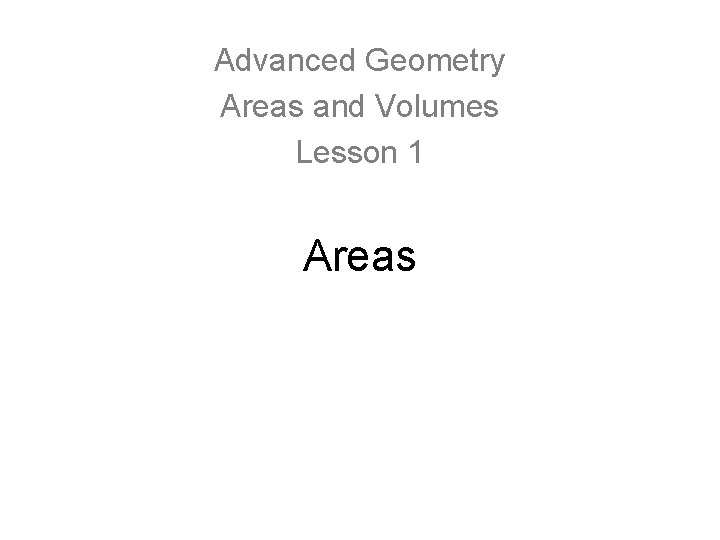Advanced Geometry Areas and Volumes Lesson 1 Areas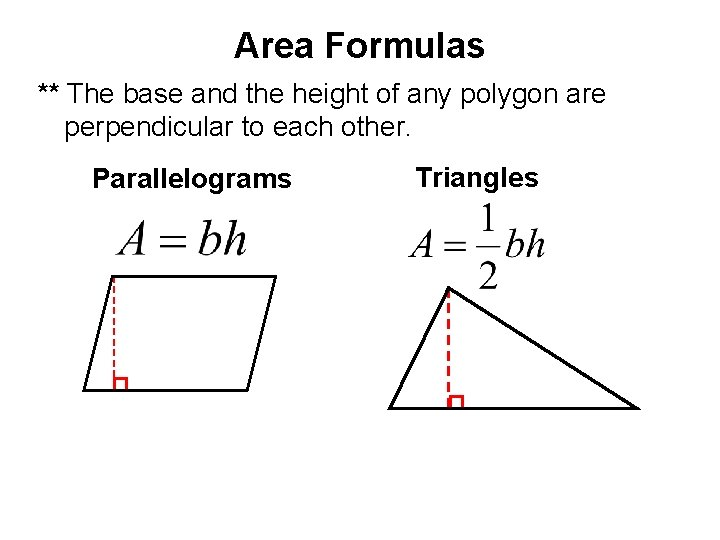Area Formulas ** The base and the height of any polygon are perpendicular to each other. Parallelograms TrianglesTrapezoids Rhombi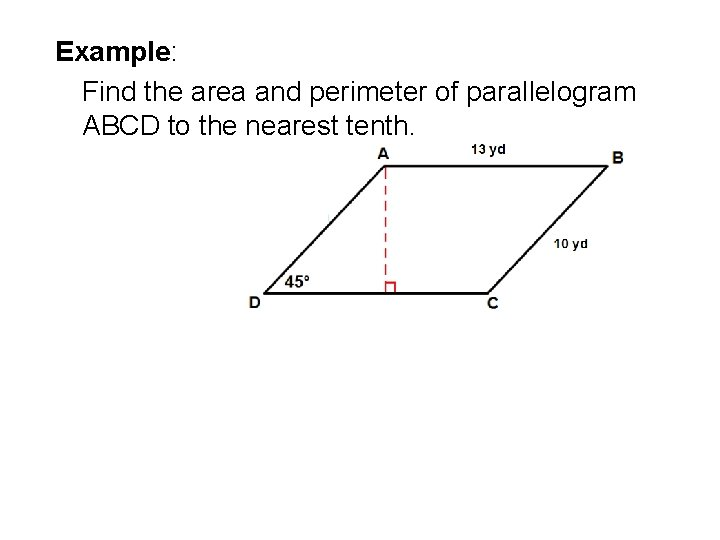Example: Find the area and perimeter of parallelogram ABCD to the nearest tenth.Example: Find the area and perimeter of parallelogram RSTU.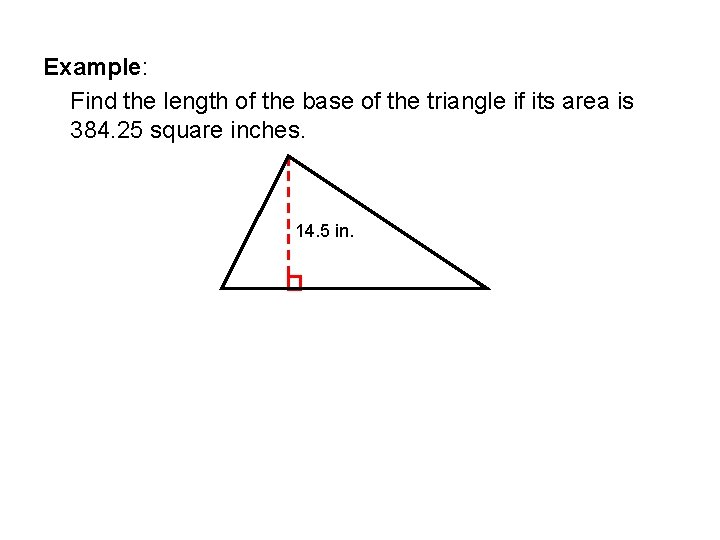Example: Find the length of the base of the triangle if its area is 384. 25 square inches. 14. 5 in.Example: Find the area of quadrilateral ABCD if AC = 35, BF = 18, and DE = 10.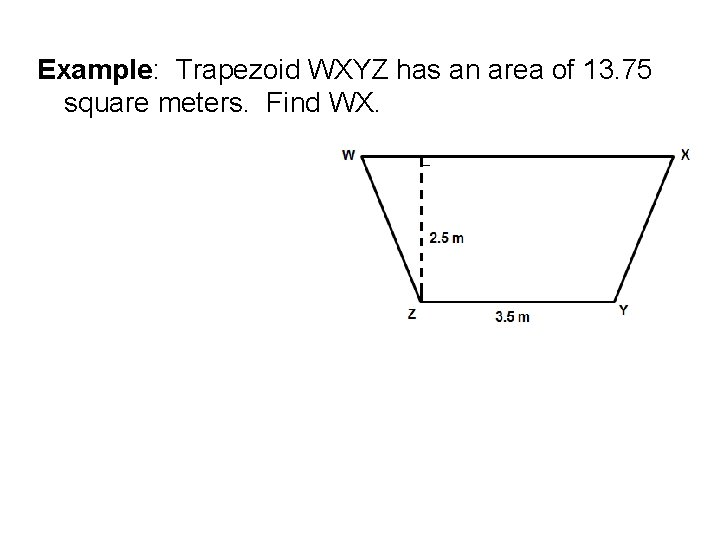Example: Trapezoid WXYZ has an area of 13. 75 square meters. Find WX.Example: Rhombus RSTU has an area of 64 square inches. Find US if RT = 8 inches.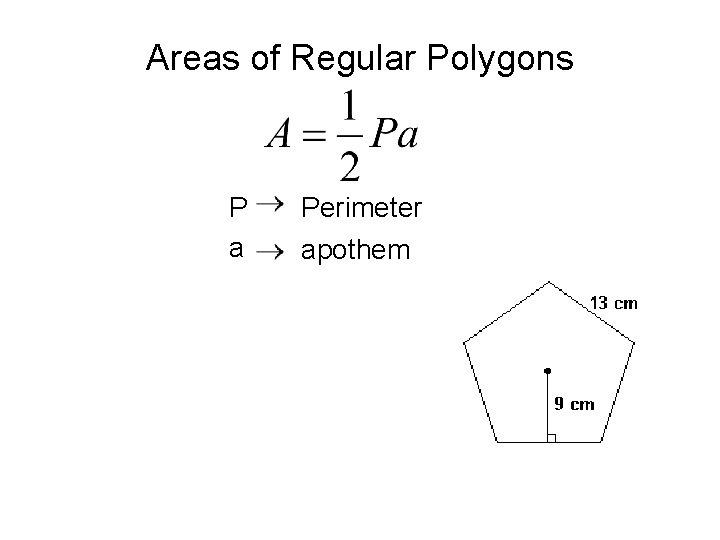Areas of Regular Polygons P a Perimeter apothemExample: Find the area of a regular pentagon with a perimeter of 90 meters to the nearest tenth.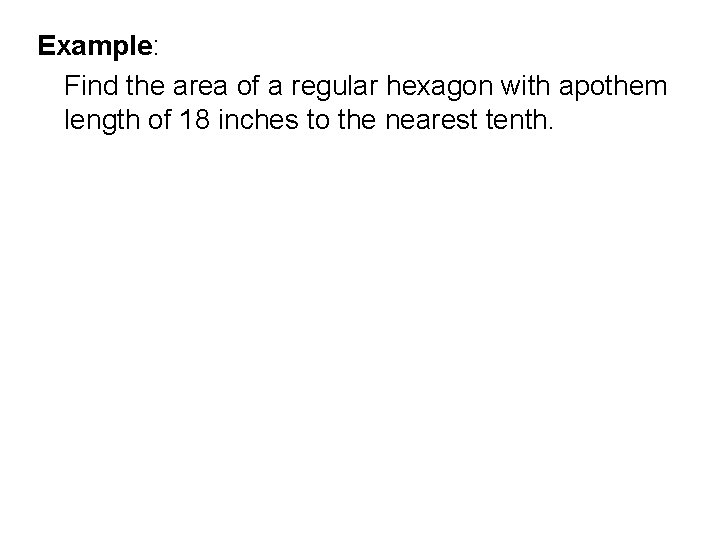Example: Find the area of a regular hexagon with apothem length of 18 inches to the nearest tenth.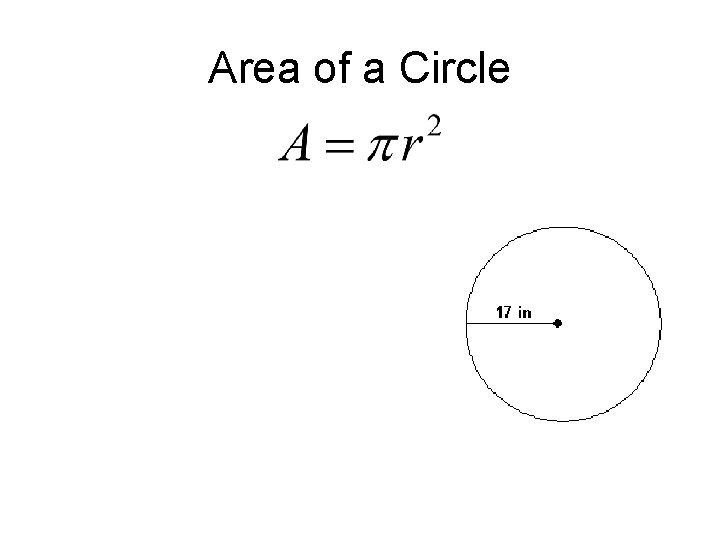Area of a Circle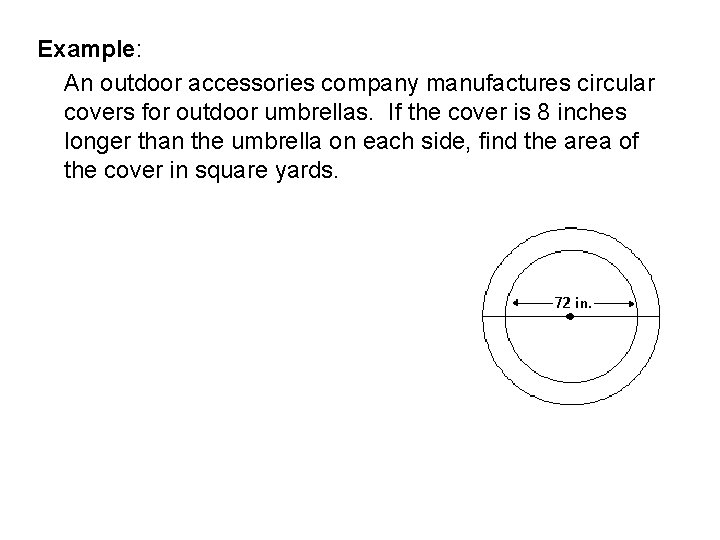Example: An outdoor accessories company manufactures circular covers for outdoor umbrellas. If the cover is 8 inches longer than the umbrella on each side, find the area of the cover in square yards.Example: Find the area of the shaded region. Assume that the quadrilateral is a square. Round to the nearest tenth. ft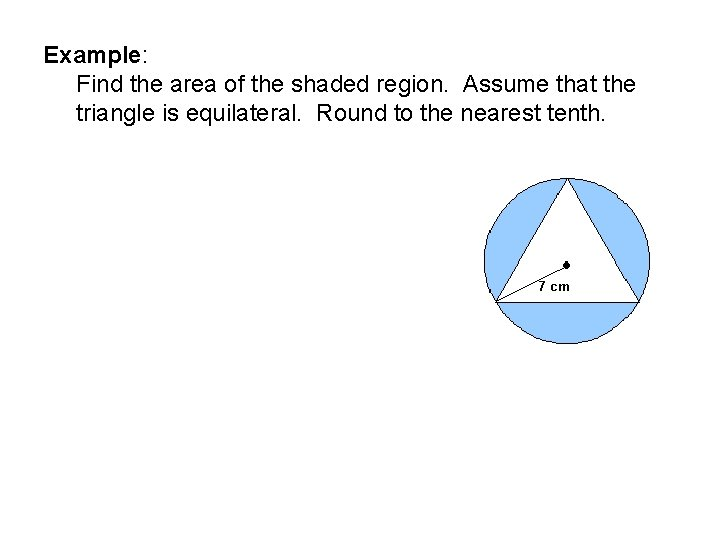Example: Find the area of the shaded region. Assume that the triangle is equilateral. Round to the nearest tenth.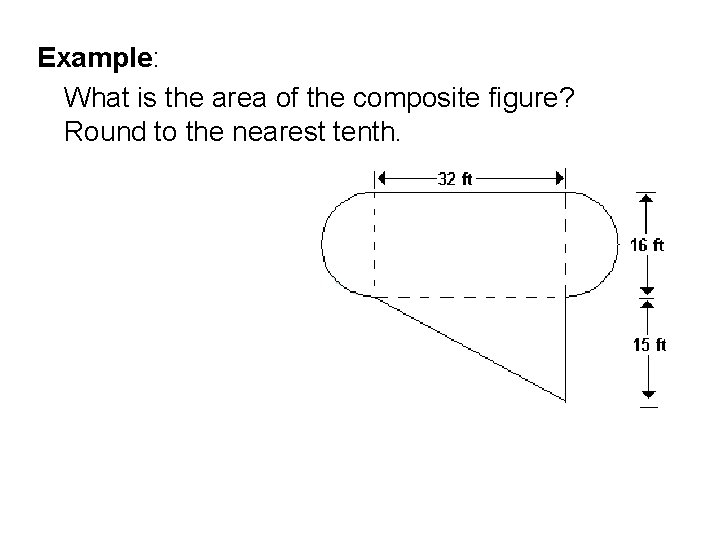Example: What is the area of the composite figure? Round to the nearest tenth.Example: Find the area of the composite figure. Round to the nearest tenth, if necessary.